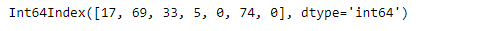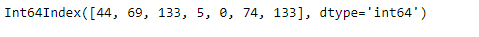GeeksforGeeks App
Open AppBrowser
Continue

# Python | Pandas Index.argmax()

Python is a great language for doing data analysis, primarily because of the fantastic ecosystem of data-centric python packages. Pandas is one of those packages and makes importing and analyzing data much easier.

Pandas` Index.argmax()` function returns the indices of the maximum value present in the input Index. If we are having more than one maximum value (i.e. maximum value is present more than once) then it returns the index of the first occurrence of the maximum value.

Syntax: Index.argmax(axis=None)

Parameter: Doesn’t take any parameter.

Example #1: Use `Index.argmax()` function to find the index of the maximum value present in the given Index.

 `# importing pandas as pd``import` `pandas as pd`` ` `# Creating the Index``df ``=` `pd.Index([``17``, ``69``, ``33``, ``5``, ``0``, ``74``, ``0``])`` ` `# Print the Index``df`

Output :Let’s find the index of the maximum value present in our Index.

 `# function to return the index ``# of the maximum value.``df.argmax()`

Output :

`5`

As we can see in the output, The maximum value in the Index is 74 and its index is 5 so the output is 5.

Example #2: Use `Index.argmax()` function to find the index of the maximum value when we are having maximum value repeated more than once.

 `# importing pandas as pd``import` `pandas as pd`` ` `# Creating the Index``df ``=` `pd.Index([``44``, ``69``, ``133``, ``5``, ``0``, ``74``, ``133``])`` ` `# Print the Index``df`

Output :Let’s find the index of the maximum value.

 `# We call the argmax() function to ``# find the index of maximum value.``df.argmax()`

Output :

`2`

As we can see in the output, The `Index.argmax()` function has returned the index of the first occurrence of the maximum value.

My Personal Notes arrow_drop_up